#### 期刊菜单

An Analysis of Fat Boundary Method with Respect to Algorithmic Parameters and an Interpolation Method for Dual Fat Boundary Method
DOI: 10.12677/IJM.2021.101005, PDF, HTML, XML, 下载: 262  浏览: 325

Abstract: Finite Element Method (FEM) has achieved a great success both in the field of academic and engi-neering. However, there are some problems for FEM. For example, the mesh generation procedure consumes a lot of time for an engineering problem to avoid mesh distortion. Some fictitious domain methods have been developed to tackle this problem. Recently, the Fat Boundary Method (FBM) has been proposed and applied to elasticity and as an improvement of FBM, the Dual Fat Boundary Method (DFBM) is proposed. These methods need an iteration procedure. In this article, the algorithmic parameters related to the iteration of FBM are studied and an interpolation method based on DFBM is proposed to reduce computational costs.

1. 引言

2. 弹性力学宽边界法与双宽边界法

$\left\{\begin{array}{l}-\nabla \cdot \sigma =f\text{\hspace{0.17em}}\text{\hspace{0.17em}}\text{in}\text{\hspace{0.17em}}\Omega \\ {u}_{|{\Gamma }_{D}}={g}_{1},\text{\hspace{0.17em}}{u}_{|{\Gamma }_{0}}={g}_{2}\\ {\left(\sigma \cdot n\right)}_{|{\Gamma }_{N}}=t\end{array}$ (1)

$\left\{\begin{array}{l}\text{I}\text{.}\text{\hspace{0.17em}}\text{\hspace{0.17em}}\left\{\begin{array}{l}-\nabla \cdot \sigma \left({u}_{1}\right)=f\text{\hspace{0.17em}}\text{\hspace{0.17em}}\text{in}\text{\hspace{0.17em}}{\Omega }_{1}\\ {u}_{1|{\Gamma }_{0}}={g}_{2},{u}_{1|{\Gamma }_{1}}={\stackrel{¯}{u}}_{|{\Gamma }_{1}}\end{array}\\ \text{II}.\text{\hspace{0.17em}}\text{\hspace{0.17em}}\left\{\begin{array}{l}-\nabla \cdot \sigma \left(\stackrel{¯}{u}\right)=\stackrel{¯}{f}+\left(\sigma \left({u}_{1}\right)\cdot n-\sigma \left({u}_{0}\right)\cdot n\right){\delta }_{{\Gamma }_{0}}\text{\hspace{0.17em}}\text{\hspace{0.17em}}\text{in}\text{\hspace{0.17em}}{\Omega }_{fict}\\ {\stackrel{¯}{u}}_{|{\Gamma }_{D}}={g}_{1},{\left(\sigma \left(\stackrel{¯}{u}\right)\cdot n\right)}_{|{\Gamma }_{N}}=t\end{array}\end{array}$ (2)

$\left\{\begin{array}{l}-\nabla \cdot \sigma \left({u}_{0}\right)=0\text{\hspace{0.17em}}\text{\hspace{0.17em}}\text{in}\text{\hspace{0.17em}}{\Omega }_{0}\\ {u}_{0|{\Gamma }_{0}}={g}_{2}\end{array}$ (3)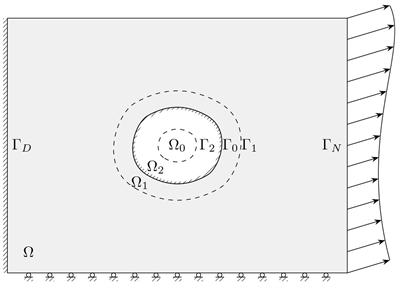Figure 1. FBM and DFBM of elasticity: problem definition

${\mathcal{T}}_{\theta }^{h}:\left(\stackrel{¯}{u},{u}_{1}\right)\to \left(\stackrel{¯}{U},{U}_{1}\right)$ (4)

$\left\{\begin{array}{l}\text{I}\text{.}\text{\hspace{0.17em}}\text{\hspace{0.17em}}\left\{\begin{array}{l}-\nabla \cdot \sigma \left({U}_{1}\right)=f\text{\hspace{0.17em}}\text{\hspace{0.17em}}\text{in}\text{\hspace{0.17em}}{\Omega }_{1}\\ {U}_{1|{\Gamma }_{0}}={g}_{2},{U}_{1|{\Gamma }_{1}}={\left(\theta {u}_{1}+\left(1-\theta \right)\stackrel{¯}{u}\right)}_{|{\Gamma }_{1}}\end{array}\\ \text{II}\text{.}\text{\hspace{0.17em}}\text{\hspace{0.17em}}\left\{\begin{array}{l}-\nabla \cdot \sigma \left(\stackrel{¯}{U}\right)=\stackrel{¯}{f}+\left(\sigma \left({U}_{1}\right)\cdot n-\sigma \left({u}_{0}\right)\cdot n\right){\delta }_{{\Gamma }_{0}}\text{\hspace{0.17em}}\text{\hspace{0.17em}}\text{in}\text{\hspace{0.17em}}{\Omega }_{fict}\\ {\stackrel{¯}{U}}_{|{\Gamma }_{D}}={g}_{1},{\left(\sigma \left(\stackrel{¯}{f}\right)\cdot n\right)}_{|{\Gamma }_{N}}=t\end{array}\end{array}$ (5)

$\left\{\begin{array}{l}\text{I}\text{.}\text{\hspace{0.17em}}\text{\hspace{0.17em}}\left\{\begin{array}{l}-\nabla \cdot \sigma \left({u}_{1}\right)=f\text{\hspace{0.17em}}\text{\hspace{0.17em}}\text{in}\text{\hspace{0.17em}}{\Omega }_{1}\\ {u}_{1|{\Gamma }_{0}}={g}_{2},{u}_{1|{\Gamma }_{1}}={\stackrel{¯}{u}}_{|{\Gamma }_{1}}\end{array}\\ \text{II}\text{.}\text{\hspace{0.17em}}\text{\hspace{0.17em}}\left\{\begin{array}{l}-\nabla \cdot \sigma \left(\stackrel{¯}{u}\right)=\stackrel{¯}{f}+\left(\sigma \left({u}_{1}\right)\cdot n-\sigma \left({u}_{2}\right)\cdot n\right){\delta }_{{\Gamma }_{0}}\text{\hspace{0.17em}}\text{\hspace{0.17em}}\text{in}\text{\hspace{0.17em}}{\Omega }_{fict}\\ {\stackrel{¯}{u}}_{|{\Gamma }_{D}}={g}_{1},{\left(\sigma \left(\stackrel{¯}{u}\right)\cdot n\right)}_{\mid {\Gamma }_{N}}=t\end{array}\\ \text{III}\text{.}\text{\hspace{0.17em}}\text{\hspace{0.17em}}\left\{\begin{array}{l}-\nabla \cdot \sigma \left({u}_{2}\right)=0\text{\hspace{0.17em}}\text{\hspace{0.17em}}\text{in}\text{\hspace{0.17em}}{\Omega }_{2}\\ {u}_{2|{\Gamma }_{0}}={g}_{2},{u}_{2|{\Gamma }_{2}}={\stackrel{¯}{u}}_{|{\Gamma }_{2}}\end{array}\end{array}$ (6)

${{\mathcal{T}}^{\prime }}_{\theta }^{h}:\left(\stackrel{¯}{u},{u}_{1},{u}_{2}\right)\to \left(\stackrel{¯}{U},{U}_{1},{U}_{2}\right)$ (7)

$\left\{\begin{array}{l}\text{I}\text{.}\text{\hspace{0.17em}}\text{\hspace{0.17em}}\left\{\begin{array}{l}-\nabla \cdot \sigma \left({U}_{1}\right)=f\text{\hspace{0.17em}}\text{\hspace{0.17em}}\text{in}\text{\hspace{0.17em}}{\Omega }_{1}\\ {U}_{1|{\Gamma }_{0}}={g}_{2},{U}_{1|{\Gamma }_{1}}={\left(\theta {u}_{1}+\left(1-\theta \right)\stackrel{¯}{u}\right)}_{|{\Gamma }_{1}}\end{array}\\ \text{II}\text{.}\text{\hspace{0.17em}}\text{\hspace{0.17em}}\left\{\begin{array}{l}-\nabla \cdot \sigma \left(\stackrel{¯}{U}\right)=\stackrel{¯}{f}+\left(\sigma \left({U}_{1}\right)\cdot n-\sigma \left({U}_{2}\right)\cdot n\right){\delta }_{{\Gamma }_{0}}\text{\hspace{0.17em}}\text{\hspace{0.17em}}\text{in}\text{\hspace{0.17em}}{\Omega }_{fict}\\ {\stackrel{¯}{U}}_{|{\Gamma }_{D}}={g}_{1},{\left(\sigma \left(\stackrel{¯}{U}\right)\cdot n\right)}_{\mid {\Gamma }_{N}}=t\end{array}\\ \text{III}\text{.}\text{\hspace{0.17em}}\text{\hspace{0.17em}}\left\{\begin{array}{l}-\nabla \cdot \sigma \left({U}_{2}\right)=0\text{\hspace{0.17em}}\text{\hspace{0.17em}}\text{in}\text{\hspace{0.17em}}{\Omega }_{2}\\ {U}_{2|{\Gamma }_{0}}={g}_{2},{U}_{2|{\Gamma }_{2}}={\left(\theta {u}_{2}+\left(1-\theta \right)\stackrel{¯}{u}\right)}_{|{\Gamma }_{2}}\end{array}\end{array}$ (8)

3. 基于双宽边界法的差值方法

4. 数值算例

${e}^{m}=\frac{{‖{u}^{m}-{u}^{a}‖}_{E}}{{‖{u}^{a}‖}_{E}}=\sqrt{\frac{{\int }_{\Omega }\left({ϵ}^{m}-{ϵ}^{a}\right):\left({\sigma }^{m}-{\sigma }^{a}\right)}{{\int }_{\Omega }{ϵ}^{a}:{\sigma }^{a}}}$ (9)

${e}_{rel}^{m+1}=\frac{|{e}^{m+1}-{e}^{m}|}{{e}^{m}}$ (10)

4.1. 孔边固定的开孔板的单轴拉伸问题

$\begin{array}{l}{u}_{r}=\frac{{T}_{x}}{8\mu r}\left\{\left(\chi -1\right)\left({r}^{2}-{R}^{2}\right)+\left[-\frac{2}{\chi }\left(\chi +1\right){R}^{2}+2{r}^{2}+\frac{2{R}^{4}}{\chi {r}^{2}}\right]\mathrm{cos}2\theta \right\}\\ {u}_{\theta }=-\frac{{T}_{x}}{8\mu r}\left\{-\frac{2}{\chi }\left(\chi -1\right){R}^{2}+2{r}^{2}-\frac{2{R}^{4}}{\chi {r}^{2}}\right\}\mathrm{sin}2\theta \end{array}$ (11)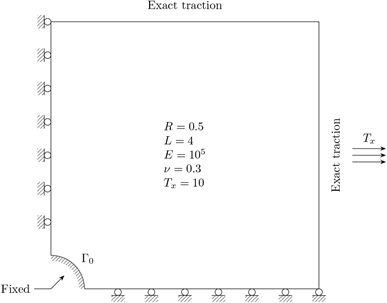Figure 2. Perforated plate with a fixed hole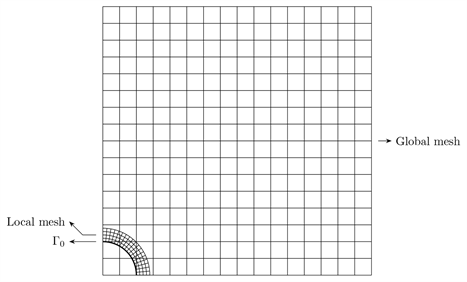Figure 3. Meshes of FBM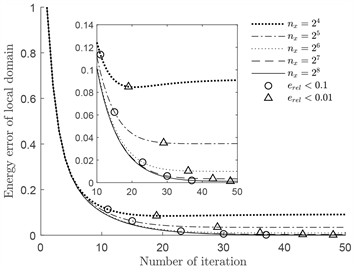Figure 4. Iteration errors for different meshes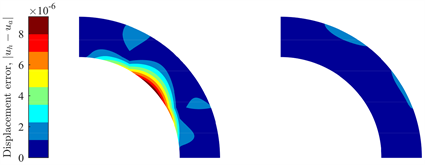Figure 5. Displacement error of global domain and local domain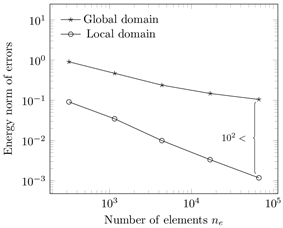Figure 6. Comparison of errors of global domain and local domain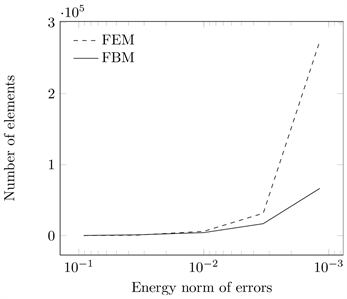Figure 7. Comparison of element numbers of FBM and FEM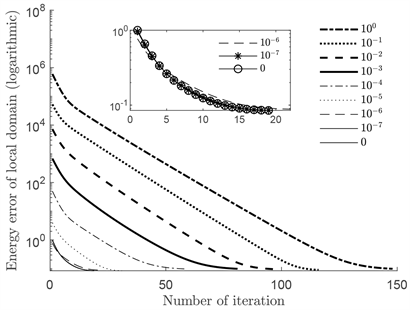Figure 8. Iteration errors for different initializations

4.2. 孔边径向位移的开孔板问题

${u}_{r}=\frac{\epsilon R}{r},\text{\hspace{0.17em}}\text{\hspace{0.17em}}{u}_{\theta }=0$ (12)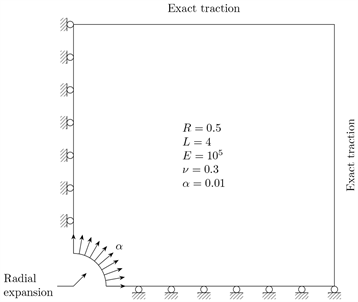Figure 9. Perforated plate with a expanded hole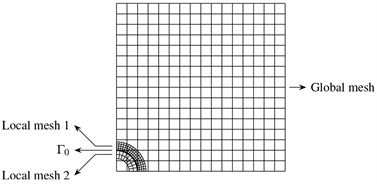Figure 10. Meshes of DFBM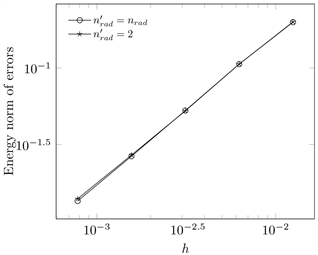Figure 11. Meshes of DFBM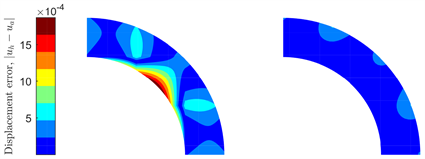Figure 12. Displacement error of global domain and local domain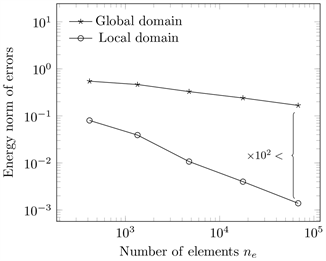Figure 13. Comparison of errors of global domain and local domain

5. 结论

NOTES

*通讯作者。

  Yagawa, G. (2011) Free Mesh Method: Fundamental Conception, Algorithms and Accuracy Study. Proceedings of the Japan Academy, Series B: Physical and Biological Sciences, 87, 115-134. https://doi.org/10.2183/pjab.87.115  Schillinger, D. and Ruess, M. (2015) The Finite Cell Method: A Review in the Context of Higher-Order Structural Analysis of CAD and Image-Based Geometric Models. Archives of Compu-tational Methods in Engineering, 22, 391-455. https://doi.org/10.1007/s11831-014-9115-y  Cottrell, J.A., Hughes, T.J.R. and Bazilevs, Y. (2009) Isogeometric Analysis: Toward Integration of CAD and FEA. Wiley, Chichester. https://doi.org/10.1002/9780470749081  Maury, B.A. (2001) Fat Boundary Method for the Poisson Problem in a Domain with Holes. Journal of Scientific Computing, 16, 319-339. https://doi.org/10.1023/A:1012821728631  Ismail, M. (2004) The Fat Boundary Method for the Numerical Resolution of Elliptic Problems in Perforated Domains. Application to 3D Fluid Flows. PhD Thesis, Universite Pierre et Marie Curie, Paris VI, France.  Vos, P.E.J., van Loon, R. and Sherwin, S.J. (2008) A Comparison of Fictitious Domain Methods Appropriate for Spectral/hp Element Discretisations. Computer Methods in Applied Mechanics and Engineering, 197, 2275-2289. https://doi.org/10.1016/j.cma.2007.11.023  de Boer, A., van Zuijlen, A.H. and Bijl, H. (2007) Review of Coupling Methods for Non-Matching Meshes. Computer Methods in Applied Mechanics and Engineering, 196, 1515-1525. https://doi.org/10.1016/j.cma.2006.03.017  Liu, K., Zhao, A. and Hu, Z. (2020) Dual Fat Boundary Method: The Fat Boundary Method in Elasticity with an Extension of the Application Scope. Mathematics and Mechanics of Solids, 26, No. 3. https://doi.org/10.1177/1081286520964499  Bertoluzza, S., Ismail, M. and Maury, B. (2011) Analysis of the Fully Discrete Fat Boundary Method. Numerical Mathematics, 18, 49-77. https://doi.org/10.1007/s00211-010-0317-4  Muskhelishvili, N.I. (1977) Some Basic Problems of the Math-ematical Theory of Elasticity. 2nd Ed., Springer, Dordrecht. https://doi.org/10.1007/978-94-017-3034-1